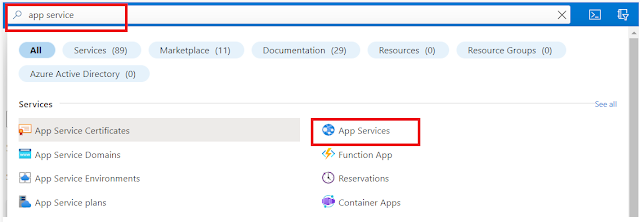### HackerRank Python Solution - Regex and Parsing - Re.findall() & Re.finditer()

re.findall():
• The expression re.findall() returns all the non-overlapping matches of patterns in a string as a list of strings.
``````>>> import re
>>> re.findall(r'\w','http://www.hackerrank.com/')
['h', 't', 't', 'p', 'w', 'w', 'w', 'h', 'a', 'c', 'k', 'e', 'r', 'r', 'a', 'n', 'k', 'c', 'o', 'm']
``````
re.finditer():
• The expression re.finditer() returns an iterator yielding MatchObject instances over all non-overlapping matches for the re pattern in the string.
``````import re
re.finditer(r'\w','http://www.hackerrank.com/')
<callable-iterator object at 0x0266C790>
map(lambda x: x.group(),re.finditer(r'\w','http://www.hackerrank.com/'))
['h', 't', 't', 'p', 'w', 'w', 'w', 'h', 'a', 'c', 'k', 'e', 'r', 'r', 'a', 'n', 'k', 'c', 'o', 'm']
``````
• You are given a string S. It consists of alphanumeric characters, spaces, and symbols(+,-).
• Your task is to find all S substrings containing 2 or more vowels.
• Also, these substrings must lie between consonants and contain vowels only.
Note:
• Vowels are defined as AEIOU and aeiou.
• Consonants are represented as QWRTYPSDFGHJKLZXCVBNM and qwrtypsdfghjklzxcvbnm.
Input Format:
• A single line of input containing string S.
Constraints:
• 0 < len(S) < 100
Output Format:
• Print the matched substrings in their order of occurrence on separate lines. If no match is found, print -1.
Sample Input:

``````rabcdeefgyYhFjkIoomnpOeorteeeeet
``````
Sample Output:

``````ee
Ioo
Oeo
eeeee
``````
Explanation:
• ee is located between consonant d and f.
• Ioo is located between consonant k and m.
• Oeo is located between consonant p and r.
• eeeee is located between consonant t and t.
Solution:

``````import re
S = input()
ans = re.findall(r"(?<=[^aeiou])([aeiou]{2,})(?=[^aeiou])", S, re.IGNORECASE)
if ans:
print(*ans, sep="\n")
else:
print(-1)
``````
Note: The problem statement is given by hackerrank.com but the solution is generated by the Geek4Tutorial admin. We highly recommend you solve this on your own, however, you can refer to this in case of help. If there is any concern regarding this post or website, please contact us using the contact form. Thank you!